### 8.4.1.1 Solution concepts

A feedback plan,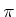, is called a solution to the problem in Formulation 8.2 if from all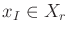, the integral curves of(considered as a vector field) arrive in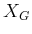, at which point the termination action is applied. Some words of caution must be given about what it means to arrive'' in. Notions of stability from control theory [523,846] are useful for distinguishing different cases; see Section 15.1. Ifis a small ball centered on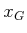, then the ball will be reached after finite time using the inward vector field shown in Figure 8.5b. Now suppose thatis a single point,. The inward vector field produces velocities that bring the state closer and closer to the origin, but when is it actually reached? It turns out that convergence to the origin in this case is only asymptotic; the origin is reached in the limit as the time approaches infinity. Such stability often arises in control theory from smooth vector fields. We may allow such asymptotic convergence to the goal (if the vector field is smooth and the goal is a point, then this is unavoidable). If any integral curves result in only asymptotic convergence to the goal, then a solution plan is called an asymptotic solution plan. Note that in general it may be impossible to require thatis a smooth (or even continuous) nonzero vector field. For example, due to the hairy ball theorem , it is known that no such vector field exists for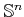for any even integer. Therefore, the strongest possible requirement is thatis smooth except on a set of measure zero; see Section 8.4.4. We may also allow solutionsfor which almost all integral curves arrive in.

However, it will be assumed by default in this chapter that a solution plan converges toin finite time. For example, if the inward field is normalized to produce unit speed everywhere except the origin, then the origin will be reached in finite time. A constraint can be placed on the set of allowable vector fields without affecting the existence of a solution plan. As in the basic motion planning problem, the speed along the path is not important. Let a normalized vector field be any vector field for which either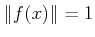or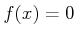, for all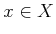. This means that all velocity vectors are either unit vectors or the zero vector, and the speed is no longer a factor. A normalized vector field provides either a direction of motion or no motion. Note that any vector field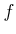can be converted into a normalized vector field by dividing the velocity vector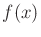by its magnitude (unless the magnitude is zero), for each.

In many cases, unit speed does not necessarily imply a constant speed in some true physical sense. For example, if the robot is a floating rigid body, there are many ways to parameterize position and orientation. The speed of the body is sensitive to this parameterization. Therefore, other constraints may be preferable instead of; however, it is important to keep in mind that the constraint is imposed so thatprovides a direction at. The particular magnitude is assumed unimportant.

So far, consideration has been given only to a feasible feedback motion planning problem. An optimal feedback motion planning problem can be defined by introducing a cost functional. Let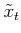denote the function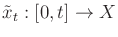, which is called the state trajectory (or state history). This is a continuous-time version of the state history, which was defined previously for problems that have discrete stages. Similarly, let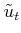denote the action trajectory (or action history),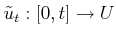. Let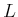denote a cost functional, which may be applied from any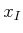to yield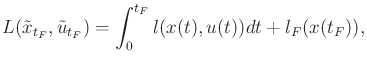(8.39)

in which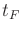is the time at which the termination action is applied. The term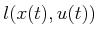can alternatively be expressed as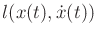by using the state transition equation (8.38). A normalized vector field that optimizes (8.39) from all initial states that can reach the goal is considered as an optimal feedback motion plan.

Note that the state trajectory can be determined from an action history and initial state. In fact, we could have used action trajectories to define a solution path to the motion planning problem of Chapter 4. Instead, a solution was defined there as a path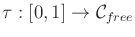to avoid having to introduce velocity fields on smooth manifolds. That was the only place in the book in which the action space seemed to disappear, and now you can see that it was only hiding to avoid inessential notation.

Steven M LaValle 2020-08-14